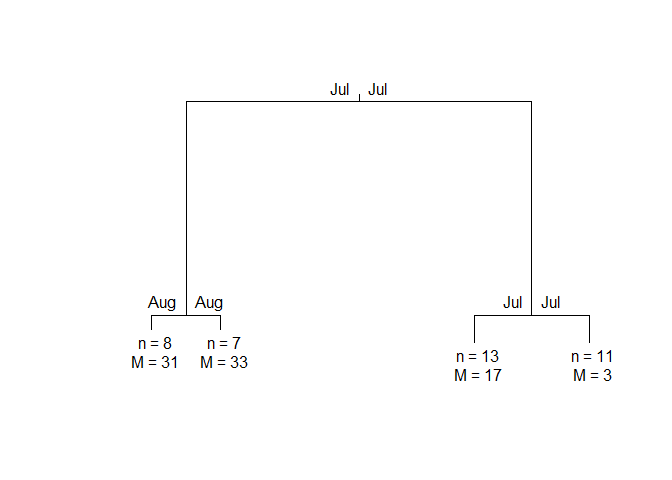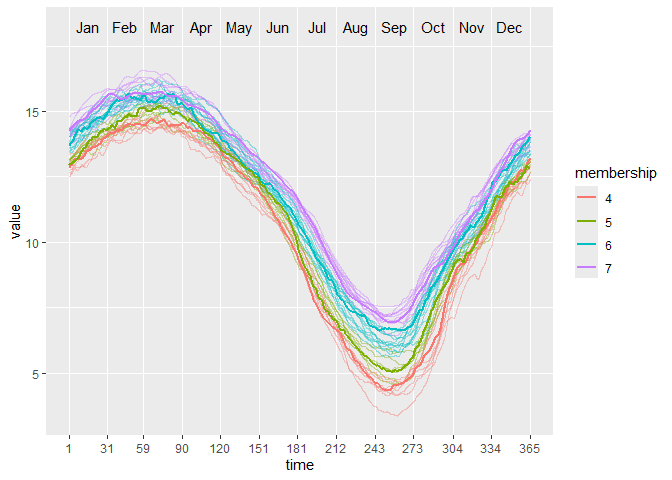Partitioning using local subregions (PULS) is a clustering technique designed to explore subregions of functional data for information to split the curves into clusters.

## Installation

You can install the released version of puls from CRAN with:

install.packages("puls")

And the development version from GitHub with:

# install.packages("remotes")
remotes::install_github("vinhtantran/puls")

## Example

This is a basic example which shows you how to solve a common problem:

library(puls)
library(fda)
#>
#> Attaching package: 'fda'
#> The following object is masked from 'package:graphics':
#>
#>     matplot
# Build a simple fd object from already smoothed smoothed_arctic
data(smoothed_arctic)
NBASIS <- 300
NORDER <- 4
y <- t(as.matrix(smoothed_arctic[, -1]))
splinebasis <- create.bspline.basis(rangeval = c(1, 365),
nbasis = NBASIS,
norder = NORDER)
fdParobj <- fdPar(fdobj = splinebasis,
Lfdobj = 2,
# No need for any more smoothing
lambda = .000001)
yfd <- smooth.basis(argvals = 1:365, y = y, fdParobj = fdParobj)

Jan <- c(1, 31); Feb <- c(31, 59); Mar <- c(59, 90)
Apr <- c(90, 120); May <- c(120, 151); Jun <- c(151, 181)
Jul <- c(181, 212); Aug <- c(212, 243); Sep <- c(243, 273)
Oct <- c(273, 304); Nov <- c(304, 334); Dec <- c(334, 365)

intervals <-
rbind(Jan, Feb, Mar, Apr, May, Jun, Jul, Aug, Sep, Oct, Nov, Dec)

PULS4_pam <- PULS(toclust.fd = yfd$fd, intervals = intervals, nclusters = 4, method = "pam") PULS4_pam #> n = 39 #> #> Node) Split, N, Cluster Inertia, Proportion Inertia Explained, #> * denotes terminal node #> #> 1) root 39 8453.2190 0.7072663 #> 2) Jul 15 885.3640 0.8431711 #> 4) Aug 8 311.7792 * #> 5) Aug 7 178.8687 * #> 3) Jul 24 1589.1780 0.7964770 #> 6) Jul 13 463.8466 * #> 7) Jul 11 371.2143 * #> #> Note: One or more of the splits chosen had an alternative split that reduced inertia by the same amount. See "alt" column of "frame" object for details. You can make a tree plot: plot(PULS4_pam)Or, a wave plot: ggwave(toclust.fd = yfd$fd, intervals = intervals, puls = PULS4_pam)